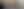Prepare for Any Job Assessment TestUpdated November 15, 2023

## Answers to Numerical Ability – Computation

1. B

2. B

3. B

4. C

5. B

6. A

7. B

8. E

9. B

10. B

11. A

12. A

Answers to Numerical Ability - Estimation

1. B

2. C

3. B

4. C

5. D

6. A

7. C

8. A

9. C

10. A

11. B

12. D

13. A

14. B

Answers to Numerical Ability - Numerical Reasoning

1. B

2. D

3. B

4. B

5. B

6. C

7. B

8. D

9. B

10. E

11. E

12. C

Answers to Numerical Ability - Data Interpretation

1. B

2. C

3. D

4. E

5. A

6. D

7. B

8. B

9. D

10. E

Answers to Verbal Ability - Spelling

1. A

2. D

3. C

4. B

5. B

6. C

7. D

8. A

9. B

10. D

11. A

12. C

13. A

14. D

15. B

16. C

17. A

18. C

19. A

20. B

If you need to prepare for a number of different employment tests and want to outsmart the competition, choose a Premium Membership from JobTestPrep.

You will get access to three PrepPacks of your choice, from a database that covers all the major test providers and employers and tailored profession packs.

Answers to Verbal Ability - Word Meaning

1. A

2. A

3. C

4. D

5. A

6. B

7. D

8. C

9. A

10. B

11. C

12. A

13. B

14. D

15. C

16. C

17. D

18. B

19. A

20. C

Answers to Verbal Ability - Word Relationship

1. A

2. D

3. B

4. D

5. C

6. C

7. D

8. B

9. C

10. A

11. C

12. B

13. A

14. C

15. D

Answers to Verbal Ability - Comprehension

1. A

2. B

3. B

4. C

5. B

6. B

7. B

8. A

9. C

10. A

Answers to Verbal Ability - Critical Reasoning

1. B

2. D

3. D

4. D

5. A

1. A

2. C

3. A

4. C

5. A

1. D

2. D

3. C

4. A

5. D

1. B

2. B

3. na

4. B

5. D

6. B

1. C

2. B

3. A

4. B

5. C

6. C

7. B

8. D

9. E

10. C